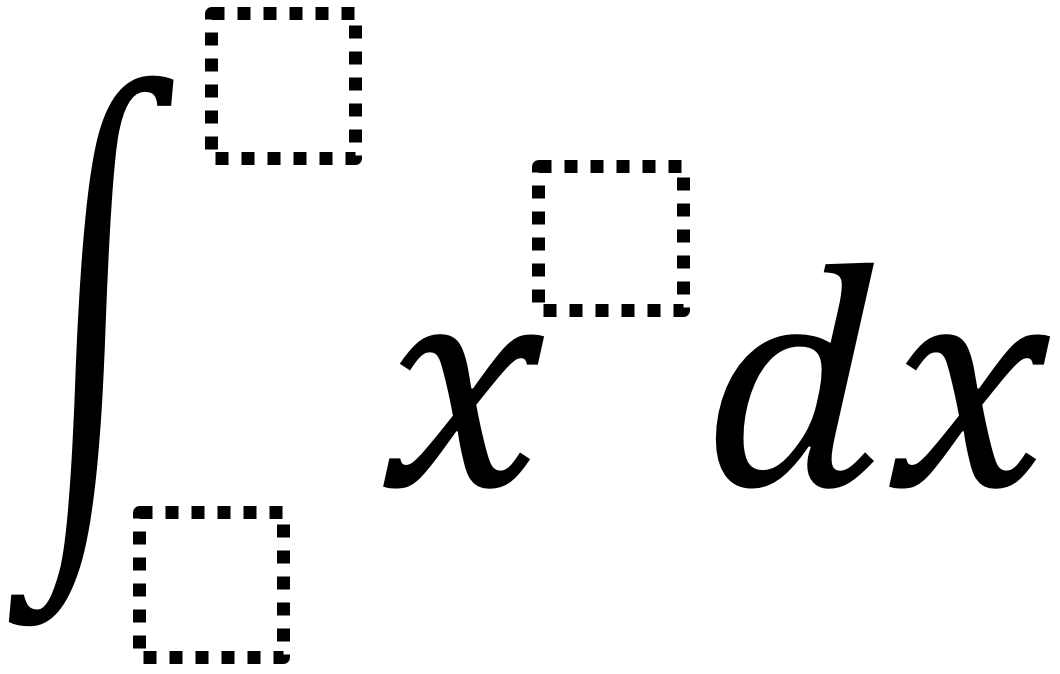Home > Calculus > Definite Integral 2

# Definite Integral 2

Directions: Use the digits 1 to 9, at most one time each, to fill in the boxes and make a positive and a negative solution.### Hint

How does the value of the x’s exponent affect the solution?
How does the placement of the digits in the upper and lower bounds affect whether the solution is positive or negative?

There are many answers. It does not matter what the value of the exponent is. The upper and lower bounds can then be any digits as long as they are not replicated. If the upper bound is larger than the lower bound, it will have a positive solution. If the lower bound is larger than the upper bound, it will have a negative solution.

Source: Robert Kaplinsky

## Derivative of Trig Functions 2

Directions: Fill in the boxes below using the digits 1 to 6, at most one …

1.•